Various types of histograms and kernel density plots of MCMC draws. See the Plot Descriptions section, below, for details.

mcmc_hist(
x,
pars = character(),
regex_pars = character(),
transformations = list(),
...,
facet_args = list(),
binwidth = NULL,
breaks = NULL,
freq = TRUE
)

mcmc_dens(
x,
pars = character(),
regex_pars = character(),
transformations = list(),
...,
facet_args = list(),
trim = FALSE
)

mcmc_hist_by_chain(
x,
pars = character(),
regex_pars = character(),
transformations = list(),
...,
facet_args = list(),
binwidth = NULL,
freq = TRUE
)

mcmc_dens_overlay(
x,
pars = character(),
regex_pars = character(),
transformations = list(),
...,
facet_args = list(),
color_chains = TRUE,
trim = FALSE
)

mcmc_dens_chains(
x,
pars = character(),
regex_pars = character(),
transformations = list(),
...,
color_chains = TRUE,
bw = NULL,
kernel = NULL,
n_dens = NULL
)

mcmc_dens_chains_data(
x,
pars = character(),
regex_pars = character(),
transformations = list(),
...,
bw = NULL,
kernel = NULL,
n_dens = NULL
)

mcmc_violin(
x,
pars = character(),
regex_pars = character(),
transformations = list(),
...,
facet_args = list(),
probs = c(0.1, 0.5, 0.9)
)

## Arguments

x A 3-D array, matrix, list of matrices, or data frame of MCMC draws. The MCMC-overview page provides details on how to specify each these allowed inputs. It is also possible to use an object with an as.array() method that returns the same kind of 3-D array described on the MCMC-overview page. An optional character vector of parameter names. If neither pars nor regex_pars is specified then the default is to use all parameters. As of version 1.7.0, bayesplot also supports 'tidy' parameter selection by specifying pars = vars(...), where ... is specified the same way as in dplyr::select(...) and similar functions. Examples of using pars in this way can be found on the Tidy parameter selection page. An optional regular expression to use for parameter selection. Can be specified instead of pars or in addition to pars. When using pars for tidy parameter selection, the regex_pars argument is ignored since select helpers perform a similar function. Optionally, transformations to apply to parameters before plotting. If transformations is a function or a single string naming a function then that function will be used to transform all parameters. To apply transformations to particular parameters, the transformations argument can be a named list with length equal to the number of parameters to be transformed. Currently only univariate transformations of scalar parameters can be specified (multivariate transformations will be implemented in a future release). If transformations is a list, the name of each list element should be a parameter name and the content of each list element should be a function (or any item to match as a function via match.fun(), e.g. a string naming a function). If a function is specified by its name as a string (e.g. "log"), then it can be used to construct a new parameter label for the appropriate parameter (e.g. "log(sigma)"). If a function itself is specified (e.g. log or function(x) log(x)) then "t" is used in the new parameter label to indicate that the parameter is transformed (e.g. "t(sigma)"). Note: due to partial argument matching transformations can be abbreviated for convenience in interactive use (e.g., transform). Currently ignored. A named list of arguments (other than facets) passed to ggplot2::facet_wrap() or ggplot2::facet_grid() to control faceting. Passed to ggplot2::geom_histogram() to override the default binwidth. Passed to ggplot2::geom_histogram() as an alternative to binwidth. For histograms, freq=TRUE (the default) puts count on the y-axis. Setting freq=FALSE puts density on the y-axis. (For many plots the y-axis text is off by default. To view the count or density labels on the y-axis see the yaxis_text() convenience function.) A logical scalar passed to ggplot2::geom_density(). Option for whether to separately color chains. Optional arguments passed to stats::density() to override default kernel density estimation parameters. n_dens defaults to 1024. A numeric vector passed to ggplot2::geom_violin()'s draw_quantiles argument to specify at which quantiles to draw horizontal lines. Set to NULL to remove the lines.

## Value

A ggplot object that can be further customized using the ggplot2 package.

## Plot Descriptions

mcmc_hist()

Histograms of posterior draws with all chains merged.

mcmc_dens()

Kernel density plots of posterior draws with all chains merged.

mcmc_hist_by_chain()

Histograms of posterior draws with chains separated via faceting.

mcmc_dens_overlay()

Kernel density plots of posterior draws with chains separated but overlaid on a single plot.

mcmc_violin()

The density estimate of each chain is plotted as a violin with horizontal lines at notable quantiles.

mcmc_dens_chains()

Ridgeline kernel density plots of posterior draws with chains separated but overlaid on a single plot. In mcmc_dens_overlay() parameters appear in separate facets; in mcmc_dens_chains() they appear in the same panel and can overlap vertically.

Other MCMC: MCMC-combos, MCMC-diagnostics, MCMC-intervals, MCMC-nuts, MCMC-overview, MCMC-parcoord, MCMC-recover, MCMC-scatterplots, MCMC-traces

## Examples

set.seed(9262017)
# some parameter draws to use for demonstration
x <- example_mcmc_draws()
dim(x)#>  250   4   4dimnames(x)#> $Iteration #> NULL #> #>$Chain
#>  "chain:1" "chain:2" "chain:3" "chain:4"
#>
#> \$Parameter
#>  "alpha"   "sigma"   "beta" "beta"
#>
##################
### Histograms ###
##################

# histograms of all parameters
color_scheme_set("brightblue")
mcmc_hist(x)#> stat_bin() using bins = 30. Pick better value with binwidth.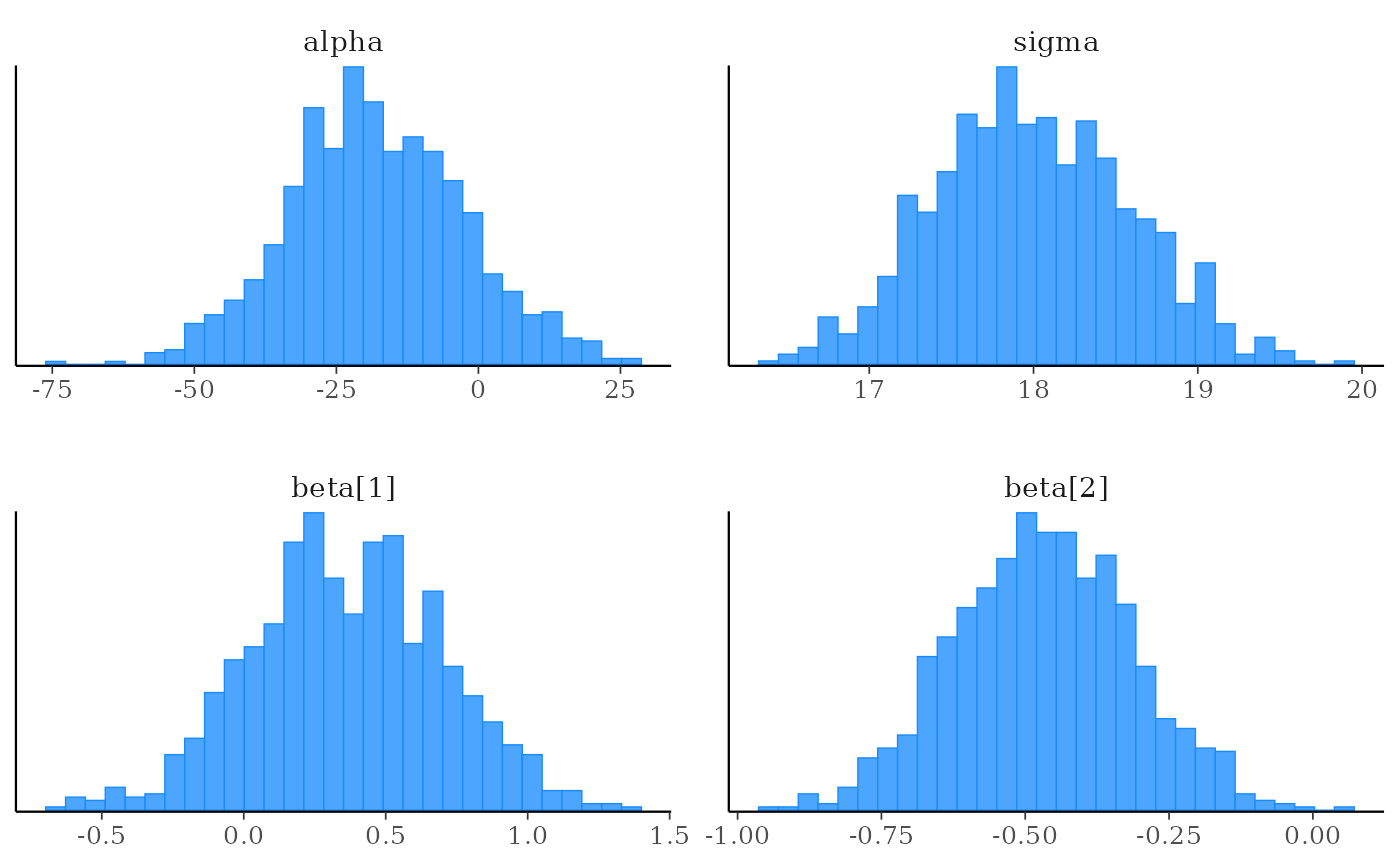# histograms of some parameters
color_scheme_set("pink")
mcmc_hist(x, pars = c("alpha", "beta"))#> stat_bin() using bins = 30. Pick better value with binwidth.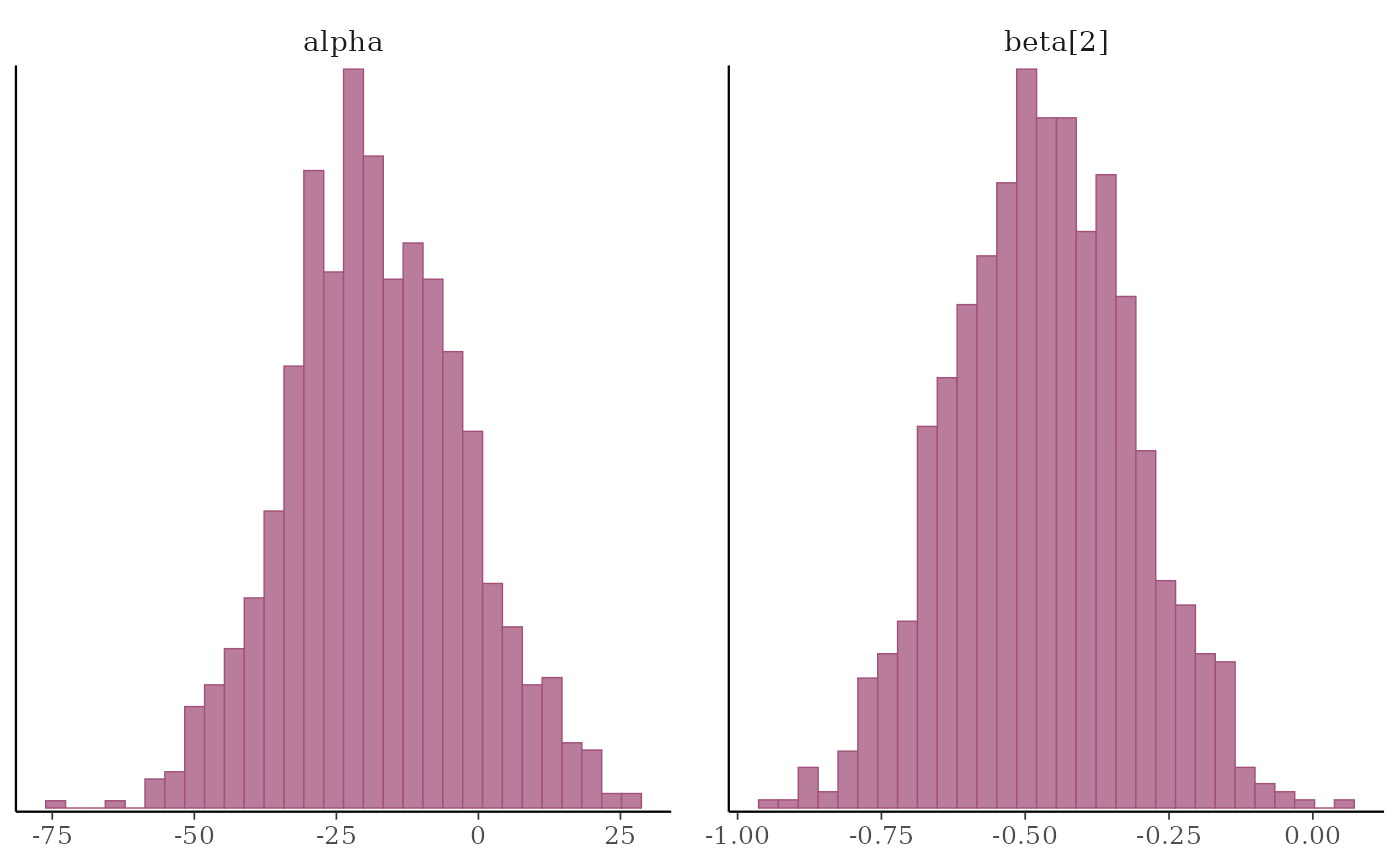# \donttest{
mcmc_hist(x, pars = "sigma", regex_pars = "beta")#> stat_bin() using bins = 30. Pick better value with binwidth.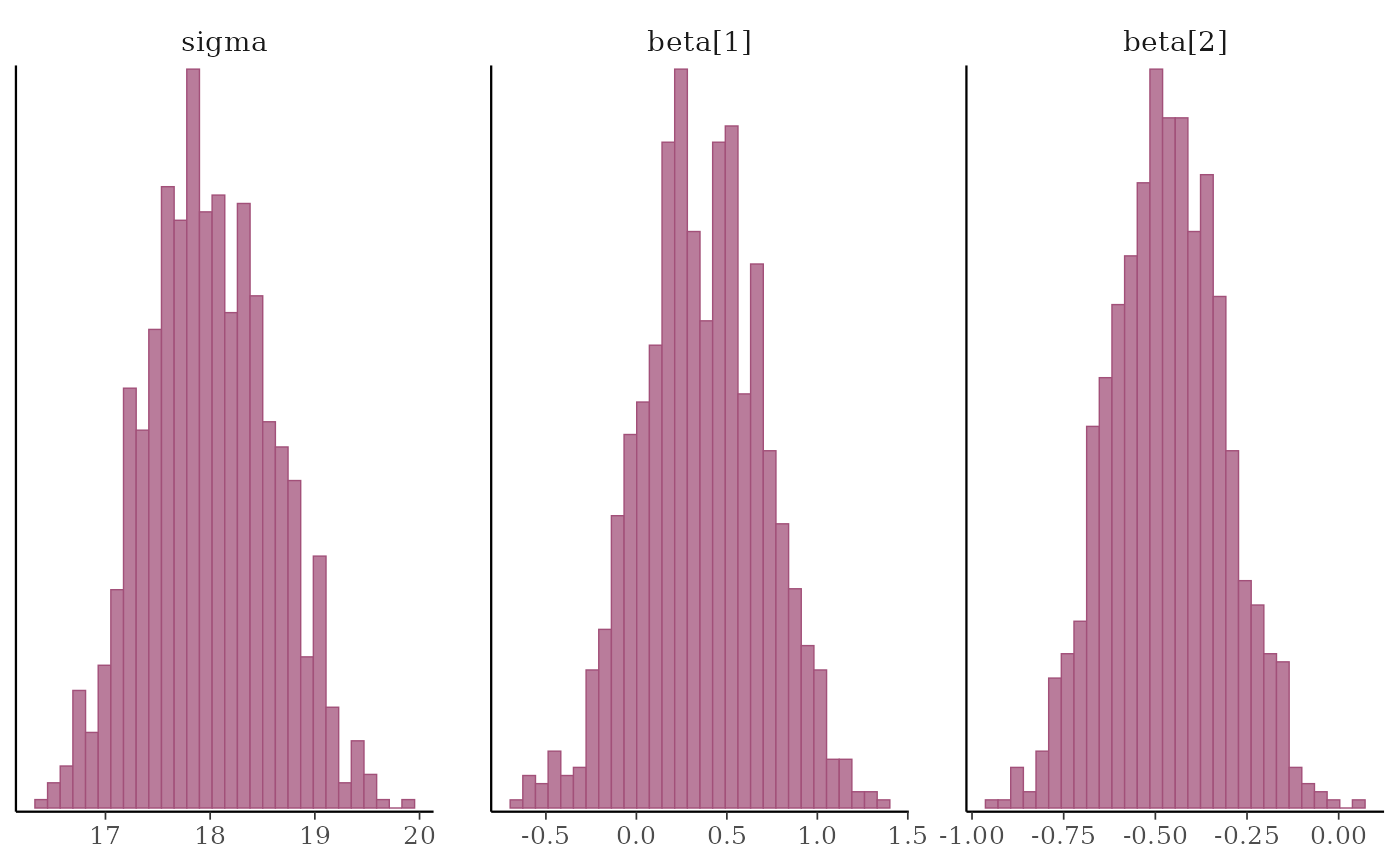# }
# example of using 'transformations' argument to plot log(sigma),
# and parsing facet labels (e.g. to get greek letters for parameters)
mcmc_hist(x, transformations = list(sigma = "log"),
facet_args = list(labeller = ggplot2::label_parsed)) +
facet_text(size = 15)#> stat_bin() using bins = 30. Pick better value with binwidth.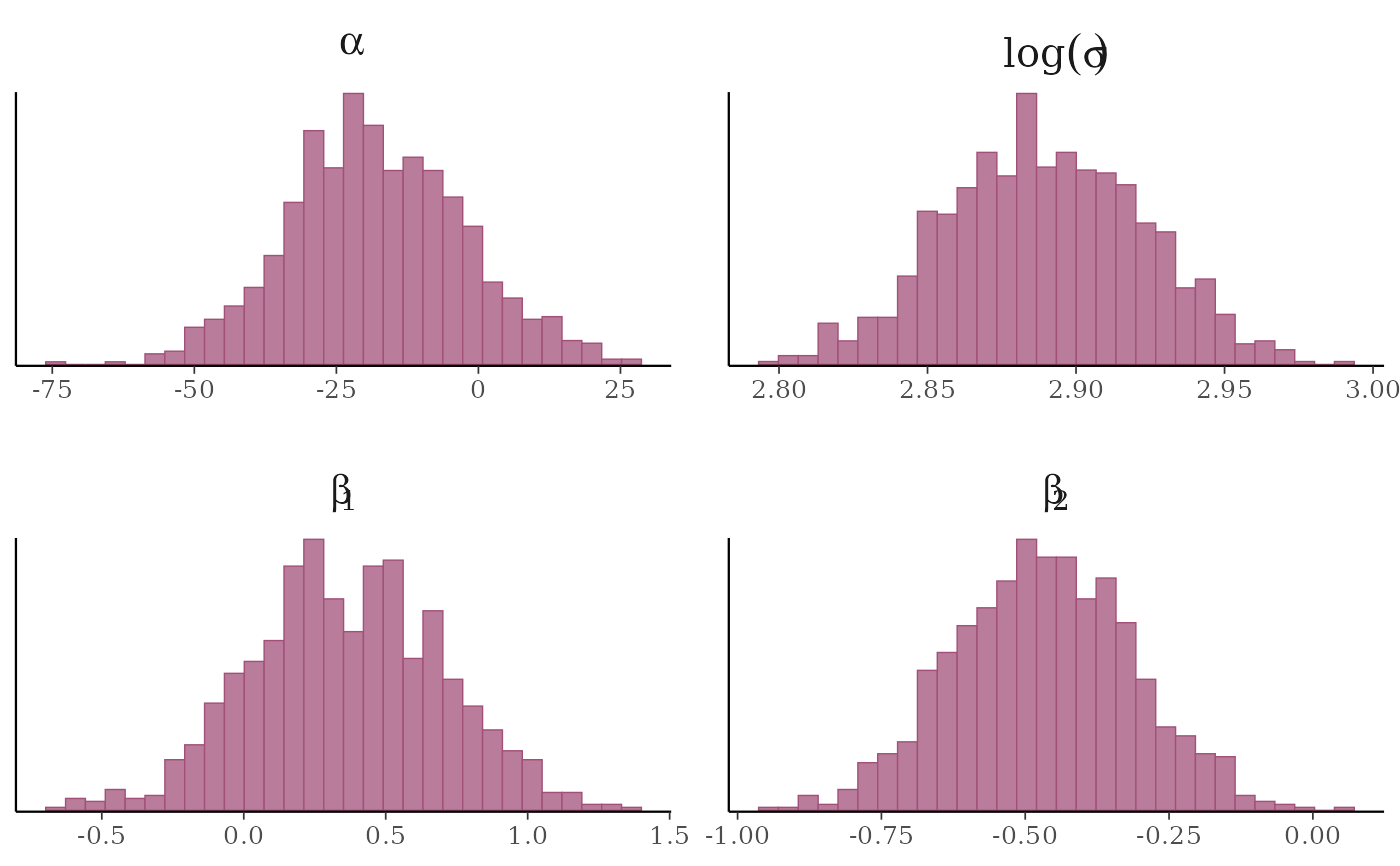# \donttest{
# instead of list(sigma = "log"), you could specify the transformation as
# list(sigma = log) or list(sigma = function(x) log(x)), but then the
# label for the transformed sigma is 't(sigma)' instead of 'log(sigma)'
mcmc_hist(x, transformations = list(sigma = log))#> stat_bin() using bins = 30. Pick better value with binwidth.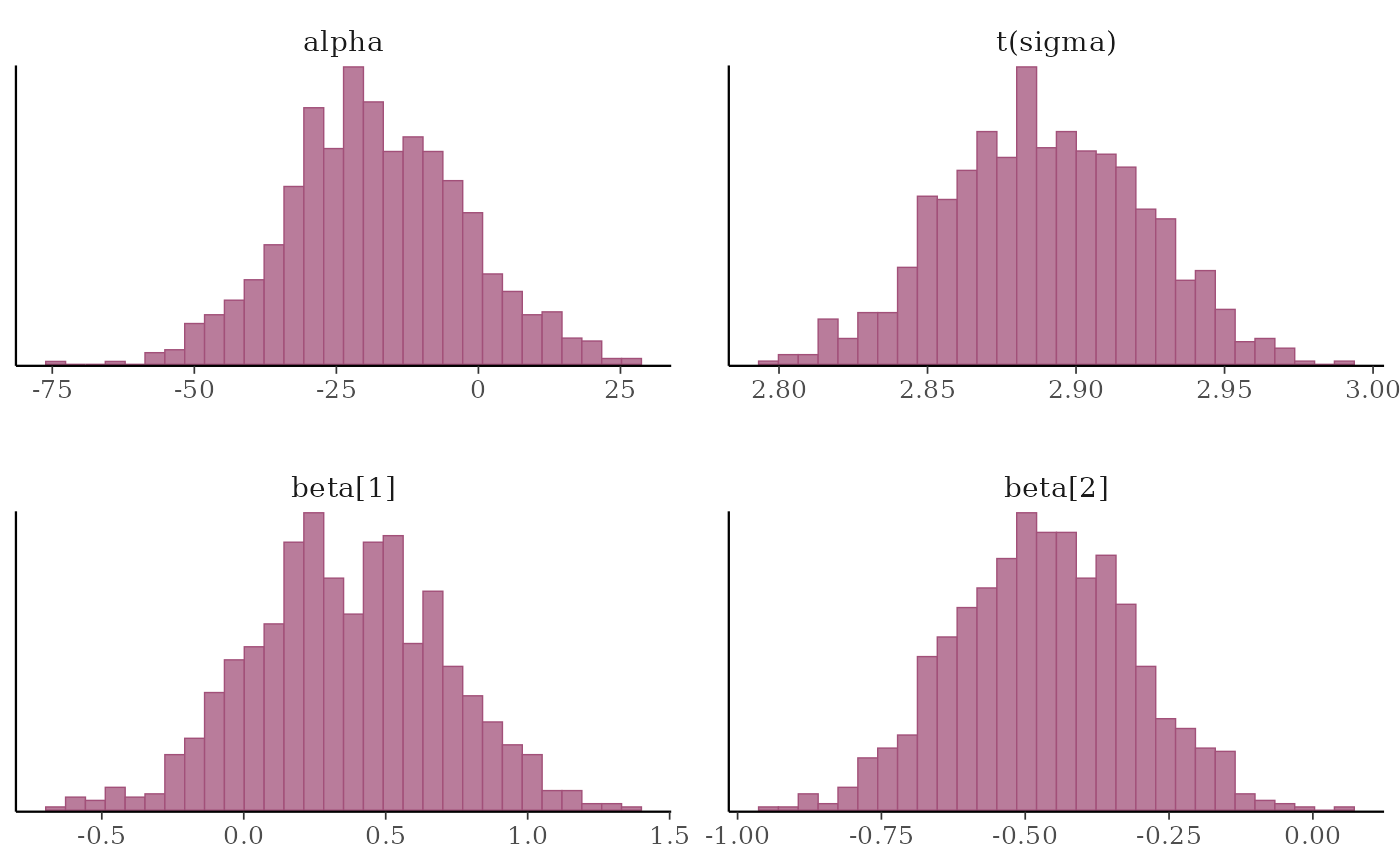# separate histograms by chain
color_scheme_set("pink")
mcmc_hist_by_chain(x, regex_pars = "beta")#> stat_bin() using bins = 30. Pick better value with binwidth.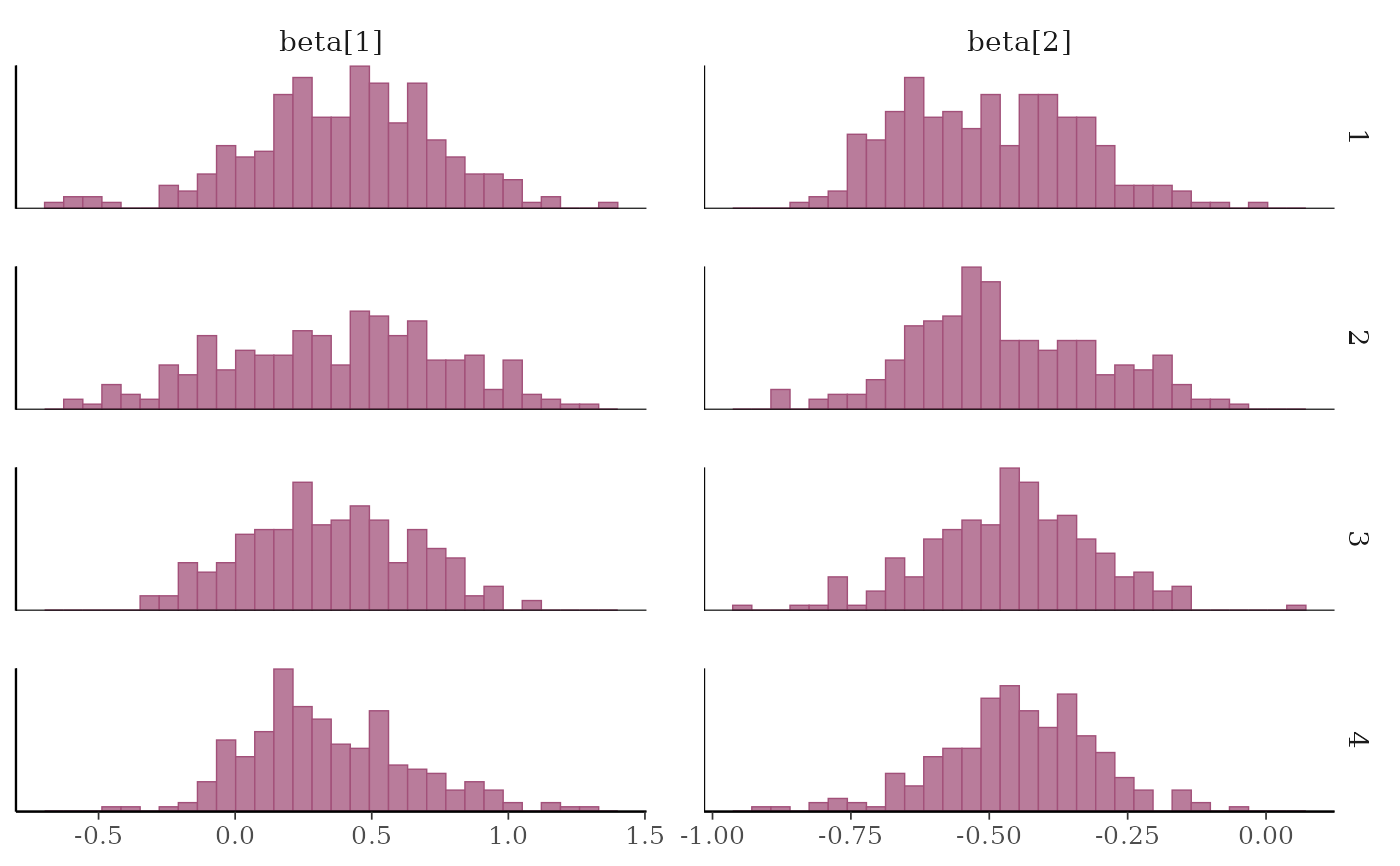# }

#################
### Densities ###
#################

mcmc_dens(x, pars = c("sigma", "beta"),
facet_args = list(nrow = 2))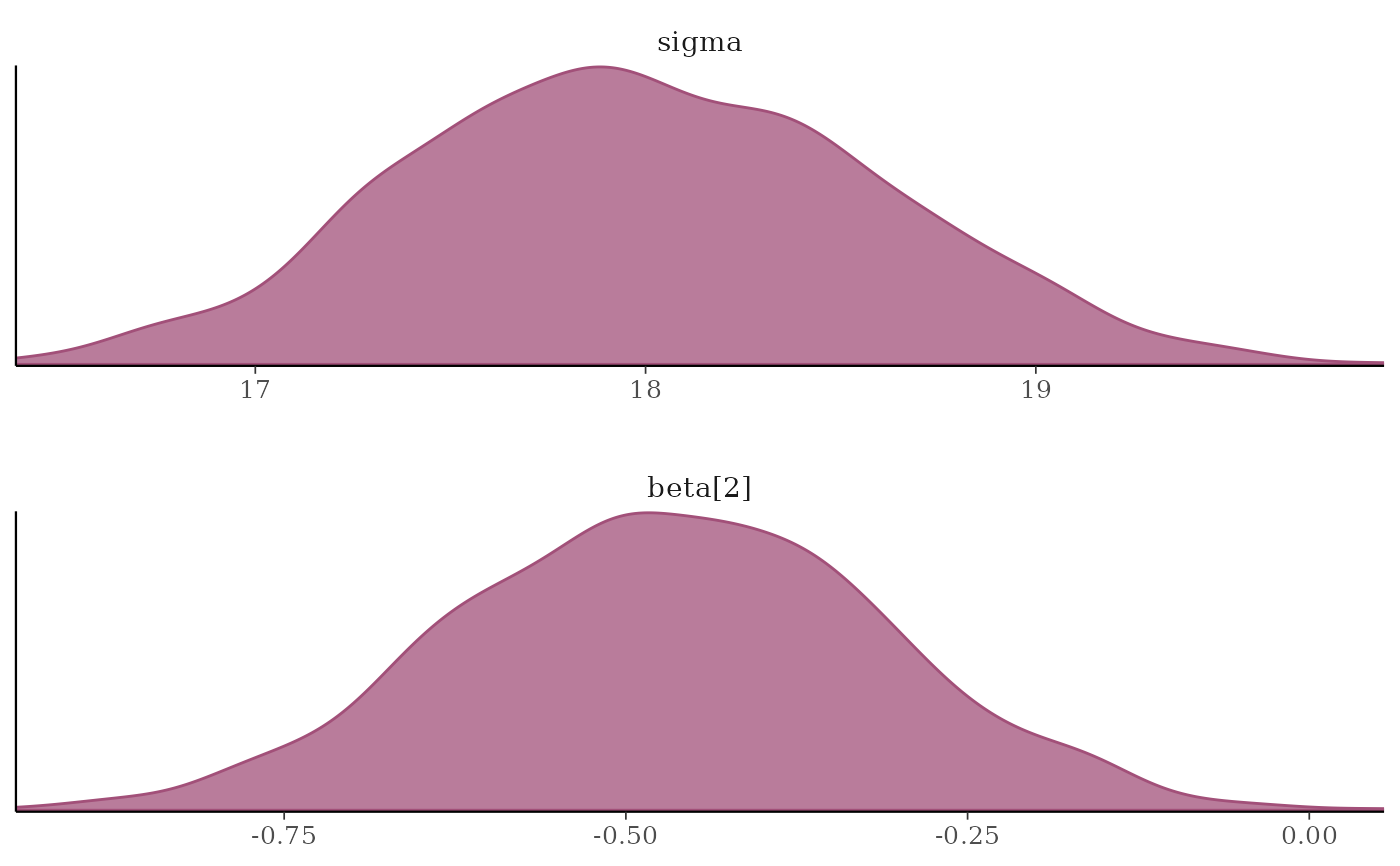# \donttest{
# separate and overlay chains
color_scheme_set("mix-teal-pink")
mcmc_dens_overlay(x, pars = c("sigma", "beta"),
facet_args = list(nrow = 2)) +
facet_text(size = 14)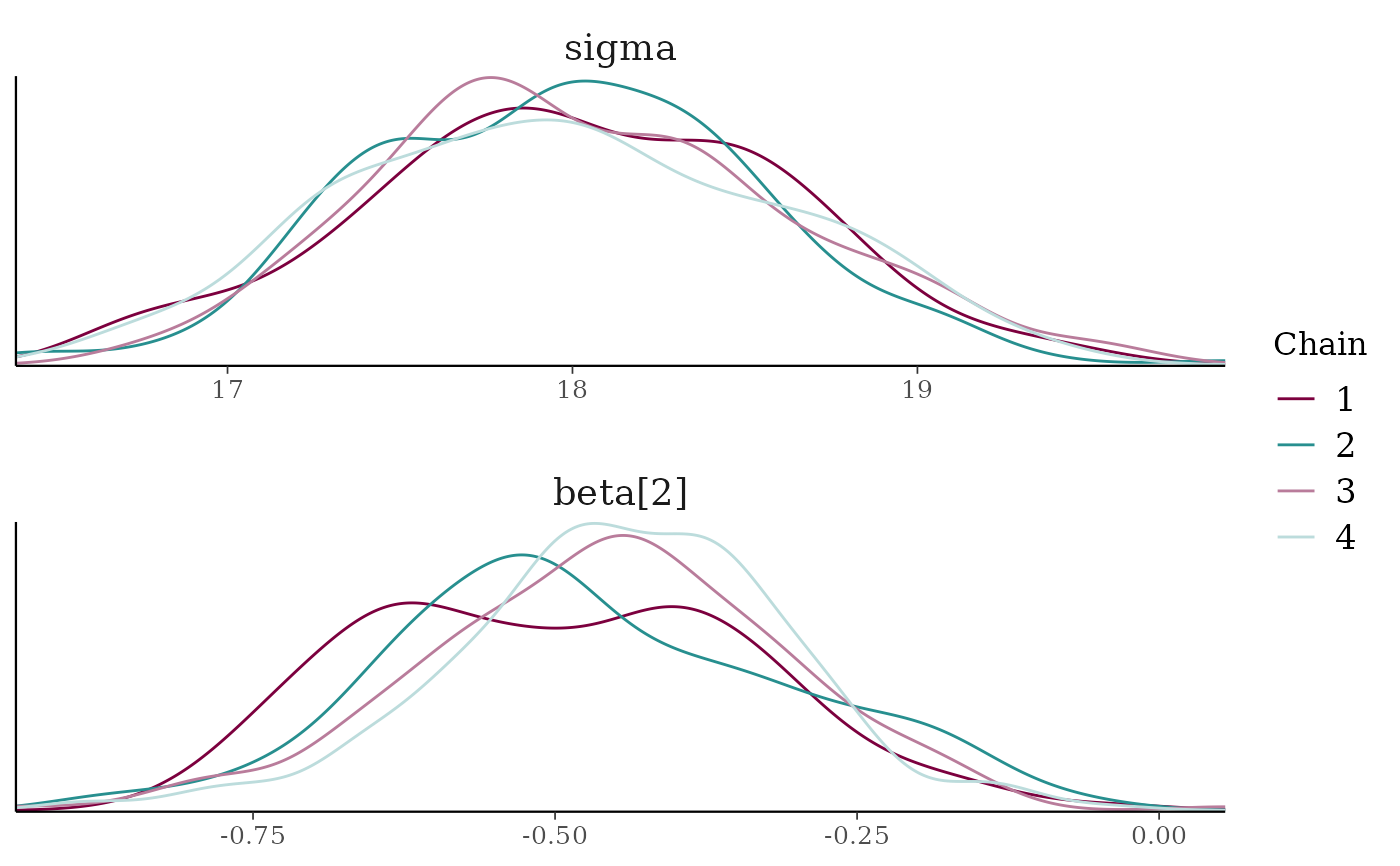x2 <- example_mcmc_draws(params = 6)
mcmc_dens_chains(x2, pars = c("beta", "beta", "beta"))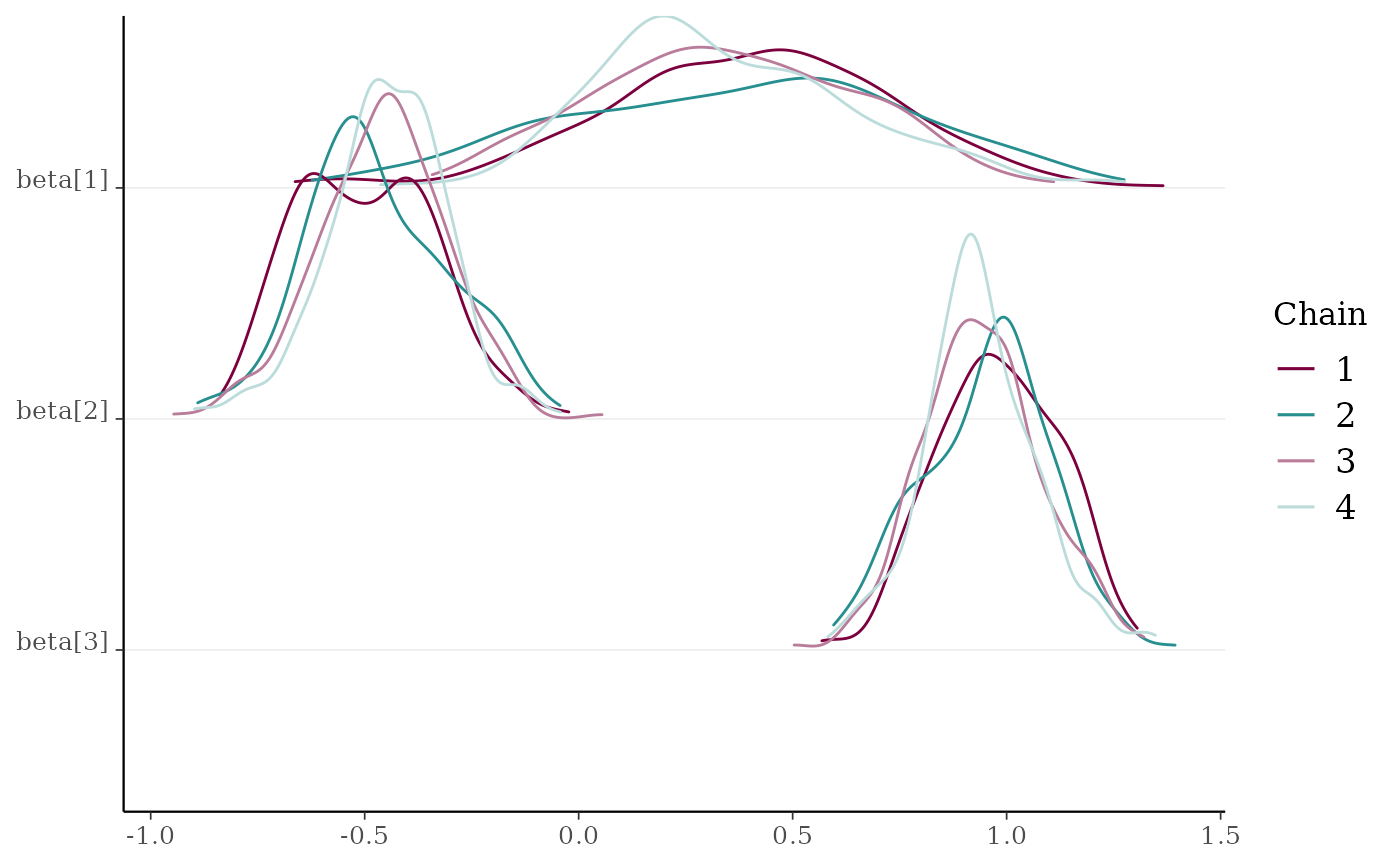# }
# separate chains as violin plots
color_scheme_set("green")
mcmc_violin(x) + panel_bg(color = "gray20", size = 2, fill = "gray30")